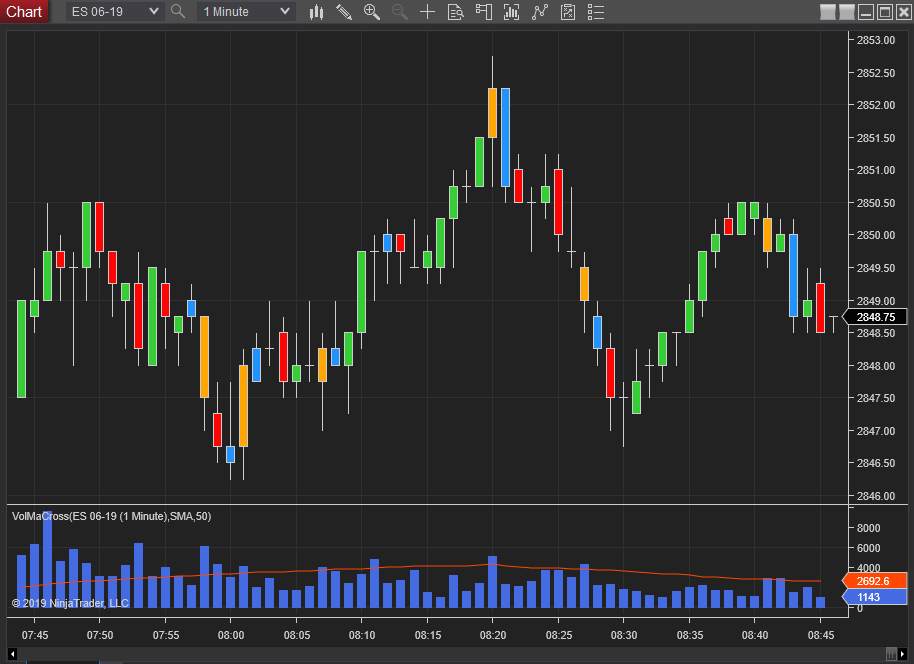## VolMACross indicator for NT8This is a conversion of the NT7 indicator Volmacross

The indicator will paint the price bar when the volume crosses above or below a user selected moving average of the volume.
The indicator plots the volume and the selected moving average of the volume in the same panel.

Options:
Moving average type of EMA, HMA, SMA, WMA
Period of the moving average.
Color for the price bar when Cross above
Color for the price bar when Cross below
Standard Plot choices for the Volume plot
Standard Plot choices for the Moving average plot